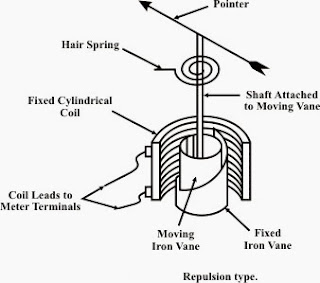# Permanent magnet moving coil( P.M.M.C.)Instrument

## Permanent magnet moving coil( P.M.M.C.)Instrument

###1. Working principle of permanent magnet moving coil

These instrument work on principle of D.C motor . According to this law when current carrying conductor lies in magnetic field then a force work current carrying conductor.
This force is directly proportional to conductor current
F,, is directly proportional to ,,I
where,, F, is force
,I, is current of conductor

### Main parts

#### 1. Permanent magnet

It is made in U shape which made of  alnico or alcomax material. it provide uniform magnetic field .

#### 2. Soft iron core

It uses between permanent magnet  and it provide support to coil . it is also provide low reluctance path for magnetic field.

#### 3. Pole shoe or Pole pieces

It only use for provide constant magnetic field to  coil.

#### 4. Magnetic coilThis coil connect with spindle and being movable . when coil  connect from supply then magnetic field developed in coil and it become movable.

#### 5. Control spring

Its work is dove-lope controlling torque in instrument. it connect with spindle.

#### 6. Damper

Air friction damping used in permanent magnet  moving coil

#### 7. Spindle

It is made from steel Rode. which connect various equipment  like as coil ,control spring , damper etc. it connect between two ball bearing .

#### 8. Pointer and Scale

It is made from Aluminum and its weight is low . In this type instrument scale being uniform.

### 3. Working principle of permanent magnet moving coilWhen we provide supply of instrument  then current will flow  in coil . which cause a magnetic field dove lope in coil and deflection torque work on coil . which deflect of moving system . By use control spring and damper generate controlling torque and damping torque when controlling torque is equal to deflecting torque then original reading show on scale.

### 4.Exasperation for various torque

1. Defection torque is directly proportional to coil current

### 5. Use of permanent magnet moving coil

#### 1. Use as an ammeter

This type instrument used as Ammeter by use resistance. A resistance connect in parallel with PMMC instrument. which called shunt resistance.

#### 2. Use as a Voltmeter

It is used as voltmeter by use of resistance . A resistance connect in  series. which called series resistance.